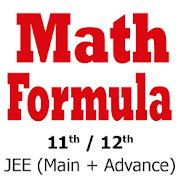# Math Formula for 11th 12thEveryone
2,208

Math Formula

This App consolidated all Math Formula required For Intermediate Student.
Extremely Useful for the students preparing for JEE main , JEE Advance , BITSAT , MHTCET , EAMCET , KCET , UPTU (UPSEE), WBJEE , VITEEE and IIT and all other Engineering Entrance Exam .
This app is also useful for those also who are preparing for Air force and NDA (national defense academy) exam.

Simple Interface : easily navigate to any topic.
Mathematical Formulas and identity equations arranged in most useful way.

Please email us at "contact.codebug@gmail.com" to add any new formulas or suggestions or topics.

The App covers topics

-- Set Theory
-- Relation and Function
-- Sequence and Series
-- Complex Number
-- De-Moviers Theorem
-- Theory of Equations
-- Statistics
-- Permutation and Combination
-- Binomial Theorem
-- Exponential and logarithmic series
-- Determinants
-- Matrices
-- Probability
-- Trigonometry Ratios
-- Trigonometric Equations
-- Solutions of Triangle
-- Inverse Trigonometric function
-- Function and Graphs
-- Limit and Continuity
-- Differential Calculus
-- Application of Derivative
-- Integration
-- Definite Integration
-- Application of Integration
-- Differential Equations
-- Co-ordinate geometry
-- Straight Line and Pair of Straight of Line
-- Circle
-- Ellipse
-- Hyperbola
-- 3 dimensional geometry
-- Straight line in space
-- The plane
-- Vector
-- Vector product
-- Triple Product of Vectors
-- Logarithm

Math Formulas - a must have app for your smartphones and tablets.

The app is continuously updated with latest details and added with new topics frequently.
Collapse

Review Policy
4.5
2,208 total
5
4
3
2
1

## What's New

Bug fixes,
general improvement.
Collapse

Updated
April 25, 2019
Size
5.3M
Installs
100,000+
Current Version
1.5
Requires Android
4.0 and up
Content Rating
Everyone
Permissions
Offered By
Codebug